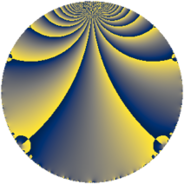# Properties

 Label 74.2.eLevel $74$ Weight $2$ Character orbit 74.e Rep. character $\chi_{74}(11,\cdot)$ Character field $\Q(\zeta_{6})$ Dimension $8$ Newform subspaces $2$ Sturm bound $19$ Trace bound $5$

# Related objects

## Defining parameters

 Level: $$N$$ $$=$$ $$74 = 2 \cdot 37$$ Weight: $$k$$ $$=$$ $$2$$ Character orbit: $$[\chi]$$ $$=$$ 74.e (of order $$6$$ and degree $$2$$) Character conductor: $$\operatorname{cond}(\chi)$$ $$=$$ $$37$$ Character field: $$\Q(\zeta_{6})$$ Newform subspaces: $$2$$ Sturm bound: $$19$$ Trace bound: $$5$$ Distinguishing $$T_p$$: $$5$$

## Dimensions

The following table gives the dimensions of various subspaces of $$M_{2}(74, [\chi])$$.

Total New Old
Modular forms 24 8 16
Cusp forms 16 8 8
Eisenstein series 8 0 8

## Trace form

 $$8 q - 4 q^{3} + 4 q^{4} - 4 q^{7} - 4 q^{9} + O(q^{10})$$ $$8 q - 4 q^{3} + 4 q^{4} - 4 q^{7} - 4 q^{9} + 4 q^{12} - 12 q^{13} - 4 q^{16} - 4 q^{21} - 8 q^{25} - 24 q^{26} + 32 q^{27} + 4 q^{28} - 12 q^{30} + 12 q^{33} + 12 q^{34} + 36 q^{35} - 8 q^{36} - 4 q^{37} - 24 q^{39} + 36 q^{42} + 12 q^{46} + 24 q^{47} + 8 q^{48} - 12 q^{49} - 12 q^{52} - 36 q^{55} - 36 q^{57} - 12 q^{58} + 36 q^{61} - 12 q^{62} + 8 q^{63} - 8 q^{64} - 12 q^{65} + 8 q^{67} - 12 q^{71} + 8 q^{73} - 24 q^{74} + 16 q^{75} - 36 q^{77} + 24 q^{78} - 12 q^{79} - 4 q^{81} - 12 q^{83} - 8 q^{84} + 24 q^{85} + 24 q^{86} - 72 q^{87} + 36 q^{89} - 24 q^{90} - 24 q^{91} - 12 q^{93} + 36 q^{94} - 12 q^{95} + 24 q^{99} + O(q^{100})$$

## Decomposition of $$S_{2}^{\mathrm{new}}(74, [\chi])$$ into newform subspaces

Label Dim $A$ Field CM Traces $q$-expansion
$a_{2}$ $a_{3}$ $a_{5}$ $a_{7}$
74.2.e.a $4$ $0.591$ $$\Q(\zeta_{12})$$ None $$0$$ $$-2$$ $$-6$$ $$-8$$ $$q+\zeta_{12}q^{2}+(\zeta_{12}-\zeta_{12}^{2}+\zeta_{12}^{3})q^{3}+\cdots$$
74.2.e.b $4$ $0.591$ $$\Q(\zeta_{12})$$ None $$0$$ $$-2$$ $$6$$ $$4$$ $$q+\zeta_{12}q^{2}+(-\zeta_{12}-\zeta_{12}^{2}-\zeta_{12}^{3})q^{3}+\cdots$$

## Decomposition of $$S_{2}^{\mathrm{old}}(74, [\chi])$$ into lower level spaces

$$S_{2}^{\mathrm{old}}(74, [\chi]) \cong$$ $$S_{2}^{\mathrm{new}}(37, [\chi])$$$$^{\oplus 2}$$